Main Content

# ecdf

Empirical cumulative distribution function

## Syntax

``````[f,x] = ecdf(y)``````
``````[f,x] = ecdf(y,Name,Value)``````
``````[f,x,flo,fup] = ecdf(___)``````
``ecdf(___)``
``ecdf(ax,___)``

## Description

example

``````[f,x] = ecdf(y)``` returns the empirical cumulative distribution function (cdf), `f`, evaluated at the points in `x`, using the data in the vector `y`.In survival and reliability analysis, this empirical cdf is called the Kaplan-Meier estimate. And the data might correspond to survival or failure times.```

example

``````[f,x] = ecdf(y,Name,Value)``` returns the empirical function values, `f`, evaluated at the points in `x`, with additional options specified by one or more `Name,Value` pair arguments.For example, you can specify the type of function to evaluate or which data is censored.```

example

``````[f,x,flo,fup] = ecdf(___)``` also returns the 95% lower and upper confidence bounds for the evaluated function values. You can use any of the input arguments in the previous syntaxes.`ecdf` computes the confidence bounds using Greenwood's formula. They are not simultaneous confidence bounds.```

example

````ecdf(___)` draws a stairstep graph of the evaluated function by using the `stairs` function. Specify `'Bounds','on'` to include the confidence bounds in the graph.```
````ecdf(ax,___)` plots on the axes specified by `ax` instead of the current axes (`gca`).```

## Examples

collapse all

Compute the Kaplan-Meier estimate of the cumulative distribution function (cdf) for simulated survival data.

Generate survival data from a Weibull distribution with parameters 3 and 1.

```rng('default') % for reproducibility failuretime = random('wbl',3,1,15,1);```

Compute the Kaplan-Meier estimate of the cdf for survival data.

```[f,x] = ecdf(failuretime); [f,x]```
```ans = 16×2 0 0.0895 0.0667 0.0895 0.1333 0.1072 0.2000 0.1303 0.2667 0.1313 0.3333 0.2718 0.4000 0.2968 0.4667 0.6147 0.5333 0.6684 0.6000 1.3749 ⋮ ```

Plot the estimated cdf.

`ecdf(failuretime)`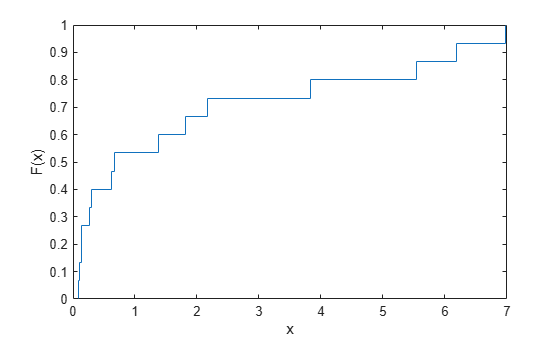Compute and plot the hazard function of simulated right-censored survival data.

Generate failure times from a Birnbaum-Saunders distribution.

```rng('default') % For reproducibility failuretime = random('birnbaumsaunders',0.3,1,100,1);```

Assuming that the end of the study is at time 0.9, generate a logical array that indicates simulated failure times that are larger than 0.9 as censored data, and store this information in a vector.

```T = 0.9; cens = (failuretime>T);```

Plot the empirical hazard function for the data.

```ecdf(failuretime,'Function','cumulative hazard', ... 'Censoring',cens,'Bounds','on');```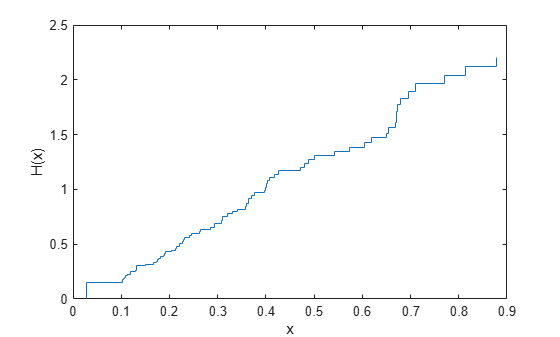Generate right-censored survival data and compare the empirical cumulative distribution function (cdf) with the known cdf.

Generate failure times from an exponential distribution with mean failure time of 15.

```rng('default') % For reproducibility y = exprnd(15,75,1);```

Generate drop-out times from an exponential distribution with mean failure time of 30.

`d = exprnd(30,75,1);`

Generate the observed failure times. They are the minimum of the generated failure times and the drop-out times.

`t = min(y,d);`

Create a logical array that indicates generated failure times that are larger than the drop-out times. The data for which this is true are censored.

`censored = (y>d);`

Compute the empirical cdf and confidence bounds.

`[f,x,flo,fup] = ecdf(t,'Censoring',censored);`

Plot the cdf and confidence bounds.

```figure() ecdf(t,'Censoring',censored,'Bounds','on'); hold on```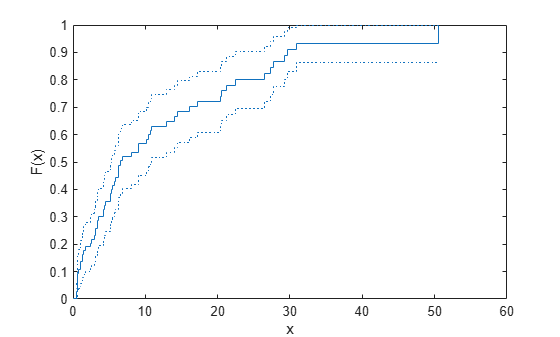Superimpose a plot of the known population cdf.

```xx = 0:.1:max(t); yy = 1-exp(-xx/15); plot(xx,yy,'g-','LineWidth',2) axis([0 50 0 1]) legend('Empirical','LCB','UCB','Population', ... 'Location','southeast') hold off```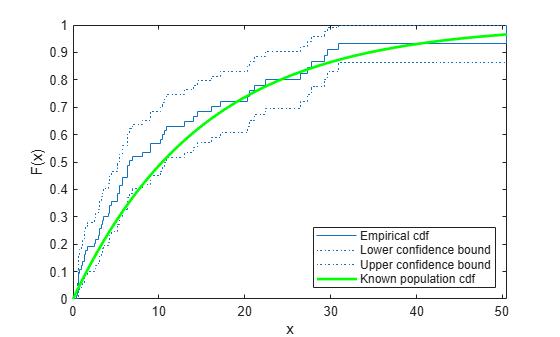Generate survival data and plot the empirical survivor function with 99% confidence bounds.

Generate lifetime data from a Weibull distribution with parameters 100 and 2.

```rng('default') % For reproducibility R = wblrnd(100,2,100,1);```

Plot the survivor function for the data with 99% confidence bounds.

```ecdf(R,'Function','survivor','Alpha',0.01,'Bounds','on') hold on```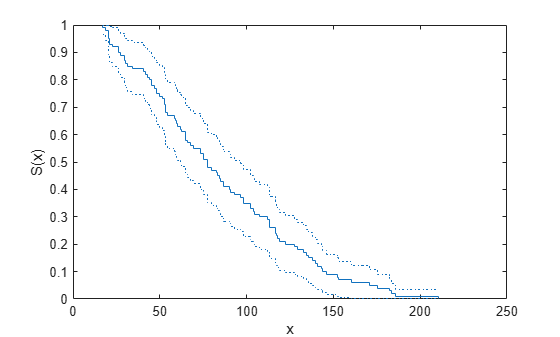Fit the Weibull survivor function.

```x = 1:1:250; wblsurv = 1-cdf('weibull',x,100,2); plot(x,wblsurv,'g-','LineWidth',2) legend('Empirical','LCB','UCB','Population', ... 'Location','northeast')```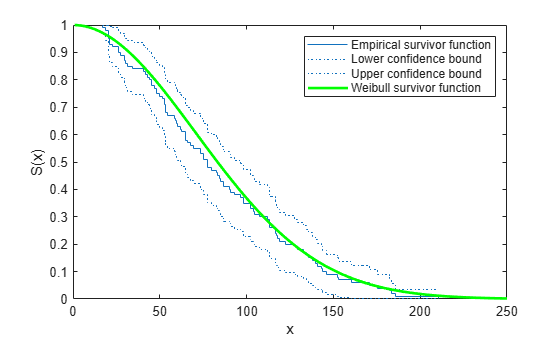The survivor function based on the actual distribution is within the confidence bounds.

## Input Arguments

collapse all

Input data, specified as a vector. For example, in survival or reliability analysis, data might be survival or failure times for each item or individual.

`ecdf` ignores `NaN` values in `y`. Additionally, any `NaN` values in the censoring vector (`'Censoring'`) or frequency vector (`'Frequency'`) cause `ecdf` to ignore the corresponding values in `y`.

Data Types: `single` | `double`

Axes handle for the figure `ecdf` plots to, specified as a handle.

For instance, if `h` is a handle for a figure, then `ecdf` can plot to that figure as follows.

Example: `ecdf(h,x)`

### Name-Value Pair Arguments

Specify optional comma-separated pairs of `Name,Value` arguments. `Name` is the argument name and `Value` is the corresponding value. `Name` must appear inside quotes. You can specify several name and value pair arguments in any order as `Name1,Value1,...,NameN,ValueN`.

Example: ```'Censoring',c,'Function','cumulative hazard','Alpha',0.025,'Bounds','on'``` specifies that `ecdf` returns the cumulative hazard function and plots the 97.5% confidence bounds, accounting for the censored data specified by vector `c`.

Indicator of censored data, specified as the comma-separated pair including `'Censoring'` and a Boolean array of the same size as `x`. Enter `1` for observations that are right-censored and `0` for observations that are fully observed. Default is all observations are fully observed.

`ecdf` ignores any `NaN` values in this censoring vector. Additionally, any `NaN` values in `y` or the frequency vector (`'Frequency'`) cause `ecdf` to ignore the corresponding values in the censoring vector.

Example: If vector `cdata` stores the censored data information, enter `'Censoring',cdata`.

Data Types: `logical`

Frequency of observations, specified as the comma-separated pair consisting of `'Frequency'` and a vector containing nonnegative integer counts. This vector is the same size as the vector `x`. The `j`th element of this vector gives the number of times the `j`th element of `x` was observed. Default is one observation per element of `x`.

`ecdf` ignores any `NaN` values in this frequency vector. Additionally, any `NaN` values in `y` or the censoring vector (`'Censoring'`) cause `ecdf` to ignore the corresponding values in the frequency vector.

Example: If `failurefreq` is a vector of frequencies, enter `'Frequency',failurefreq`

Data Types: `single` | `double`

Significance level for the confidence interval of the evaluated function, specified as the comma-separated pair consisting of `'Alpha'` and a scalar value between in the range (0,1). Default is 0.05 for 95% confidence. For a given value `alpha`, the confidence level is `100(1-alpha)`%.

For instance, for a 99% confidence interval, you can specify the alpha value as follows.

Example: `'Alpha',0.01`

Data Types: `single` | `double`

Type of function that `ecdf` evaluates and returns, specified as the comma-separated pair consisting of `'Function'` and one of the following.

 `'cdf'` Default. Cumulative distribution function. `'survivor'` Survivor function. `'cumulative hazard'` Cumulative hazard function.

Example: `'Function','cumulative hazard'`

Indicator for including bounds, specified as the comma-separated pair consisting of `'Bounds'` and one of the following.

 `'off'` Default. Specify to omit bounds. `'on'` Specify to include bounds.

Note

This name-value argument is used only for plotting.

Example: `'Bounds','on'`

## Output Arguments

collapse all

Function values evaluated at the points in `x`, returned as a column vector.

Sorted observed points in the data vector `y`, returned as a column vector.

`ecdf` sorts `y`, removes duplicate values in the sorted `y`, and saves the results to the output `x`. The output `x` includes the minimum value of `y` as its first two values. These two values are useful for plotting the outputs of `ecdf` using the `stairs` function.

Lower confidence bound for the evaluated function, returned as a column vector. `ecdf` computes the confidence bounds using Greenwood's formula. They are not simultaneous confidence bounds.

Upper confidence bound for the evaluated function, returned as a column vector. `ecdf` computes the confidence bounds using Greenwood's formula. They are not simultaneous confidence bounds.

## More About

collapse all

### Greenwood’s Formula

Approximation for the variance of Kaplan-Meier estimator.

The variance estimate is given by

`$V\left(S\left(t\right)\right)={S}^{2}\left(t\right)\sum _{{t}_{i}`

where ri is the number at risk at time ti, and di is the number of failures at time ti.

 Cox, D. R., and D. Oakes. Analysis of Survival Data. London: Chapman & Hall, 1984.

 Lawless, J. F. Statistical Models and Methods for Lifetime Data. 2nd ed., Hoboken, NJ: John Wiley & Sons, Inc., 2003.

Download ebook